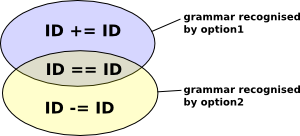# Eclipse Xtext - Syntactic Predicates

This page follows on from the xtext grammar page to discuss syntactic predicates in more detail.

Syntactic predicates allow us to resolve ambiguous grammars.

### Non-Recursive Example

 The following is an example of an ambiguous grammar. The 'Option' rule has two parts and these parts have an intersecting grammar (in this case ID == ID). This intersection is the cause of the ambiguity as it parses in both option1 and option2.Here is the complete grammar:

 ```grammar com.euclideanspace.experiment.Mydsl with org.eclipse.xtext.common.Terminals generate mydsl "https://www.euclideanspace.com/experiment/Mydsl" Model: opt=Option; Option: (ID a=Option1 b=ID) | (ID d=Option2 e=ID) ; Option1: a=('=='|'+='); Option2: d=('=='|'-=');```

This is not designed to be a grammar with any practical use, it is just a test case so I can experiment with syntactic predicates. I have included assignments (such as 'a=') so that I know which option is taken (I edited the labelProvider file to give me information about this).

As it stands the grammar is ambiguous and it has no syntactic predicates so it gives the following warnings:

 ```warning(200): ../com.euclideanspace.experiment/src-gen/com/euclideanspace/experiment/parser/antlr/internal/InternalMydsl.g:119:1: Decision can match input such as "RULE_ID '==' RULE_ID" using multiple alternatives: 1, 2 As a result, alternative(s) 2 were disabled for that input warning(200): ../com.euclideanspace.experiment.ui/src-gen/com/euclideanspace/experiment/ui/contentassist/antlr/internal/InternalMydsl.g:176:1: Decision can match input such as "RULE_ID '==' RULE_ID" using multiple alternatives: 1, 2 As a result, alternative(s) 2 were disabled for that input```

The grammar is ambiguous because input like 'a == b' can match using either Option1 or Option2.

We can remove this warning by adding a syntactic predicate indicated by '=>' before the option that we want to choose for the potentially ambiguous input.
 ```Option: =>(ID a=Option1 b=ID) | (ID d=Option2 e=ID) ;```

I now want to experiment by putting the syntactic predicate in various places in the 'Option' rule and finding out how input is parsed in each case:

Option Rule Input Option Chosen
```Option:
=>(ID a=Option1 b=ID)
|
(ID d=Option2 e=ID)
;```
x == y Option1
x -= y Option2
x += y Option1
```Option:
(=>ID a=Option1 b=ID)
|
(ID d=Option2 e=ID)
;```
x == y Option1
x -= y Option2
x += y Option1
```Option:
(ID =>a=Option1 b=ID)
|
(ID d=Option2 e=ID)
;```
compiler warning
```Option:
(ID a=Option1 =>(b=ID))
|
(ID d=Option2 e=ID)
;```
compiler warning
```Option:
(ID a=Option1 b=ID)
|
=>(ID d=Option2 e=ID)
;```
x == y Option1
x -= y Option2
x += y Option1
```Option:
(ID a=Option1 b=ID)
|
(=>ID d=Option2 e=ID)
;```
x == y Option1
x -= y Option2
x += y Option1
```Option:
(ID a=Option1 b=ID)
|
(ID =>(d=Option2) e=ID)
;```
compiler warning
```Option:
(ID a=Option1 b=ID)
|
(ID d=Option2 =>(e=ID))
;```
compiler warning

We can see that for the ambiguous case (x == y) the option that appears first in the rule is the option chosen.

In Antlr3 the production to the right of the predicate is attempted if and only if the syntactic predicate first accepts the next portion of the input stream. Although ordered, the predicates are checked first, with parsing of a clause continuing if and only if the predicate is satisfied

We could also put the syntactic predicate inside the bracket like this:
 ```Option: (ID Option1 ID) | (=>ID Option2 ID) ; Option1: '=='|'+='; Option2: '=='|'-=';```
However, if we put the syntactic predicate before Option2 (which would appear to make sense as this is the option we want to choose) then we get the warning below:
 ```Option: (ID Option1 ID) | (ID =>Option2 ID) ; Option1: '=='|'+='; Option2: '=='|'-=';```
 ```1273 [main] INFO clipse.emf.mwe.utils.GenModelHelper - Registered GenModel 'https://www.euclideanspace.com/experiment/Mydsl' from 'platform:/resource/com.euclideanspace.experiment/model/generated/Mydsl.genmodel' warning(200): ../com.euclideanspace.experiment/src-gen/com/euclideanspace/experiment/parser/antlr/internal/InternalMydsl.g:119:1: Decision can match input such as "RULE_ID '==' RULE_ID" using multiple alternatives: 1, 2 As a result, alternative(s) 2 were disabled for that input```

So it is not juse a case of putting the syntactic predicate before the option we want to choose. We must understand how the parser scans the grammar so we know where to cut off unwanted options.

reference